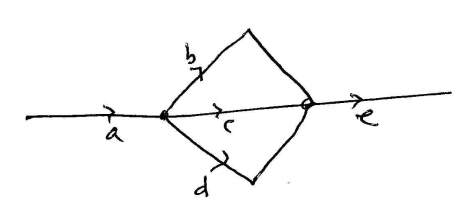# Fundamental group of complement of circle with a line passing through it.

Let $X= S^1 \cup y$-axis in the $xy$-plane. Compute the fundamental group of $\mathbb{R}^3 - X$

Edit: To clarify, $S^1 =\{(x,y)|x^2 + y^2 = 1\}$ is the unit circle in the $xy$-plane, so that the $y$-axis and the circle intersect at $(0,\pm 1).$

I tried doing this by first "blowing up" the circle and the line to get a homotopy equivalent space. It seems like when you do this blow up process you are left with a sphere with two holes in it and two line segments attached to the sphere at the endpoints of the line segment. The holes in the sphere come from $y$-axis and the line segments come from the space between the inside of the circle and the $y$-axis. Then, forgetting about the line segments for a moment we can project the rest of the space to $\mathbb{R}^2 - D^2$ via stereographic projection. The line segments are then arcs connecting two points in the plane (the arcs lying outside the plane). Finally the whole space deformation retracts to a wedge of 3 circles. Giving that $\pi_1(X) \cong \mathbb{Z}\ast\mathbb{Z}\ast\mathbb{Z}$.

This is a very visual process and I'm not sure my first space after "blowing up" is correct. Can anyone check this or give me suggestions. Also:

The space $X$ is not a knot, but can we still use the Wirtinger presentation to compute $\pi_1(X)$ by considering intersections as both over and under crossings?

Let $A=\{(x,y,z) \in X : x<\tfrac{1}{2}\}$ and $B=\{(x,y,z) \in X: x>-\tfrac{1}{2}\}$. Then $A$ and $B$ are homeomorphic and each deform retracts onto a surface $\Sigma$ surrounding "half" of the removed subspace; see below. Then $\pi_1(A)\cong \pi_1(B)\cong\pi_1(\Sigma)\cong \mathbb{Z} * \mathbb{Z} *\mathbb{Z}$. We can represent the three generators via paths as in the image below. This choice makes it clear that corresponding generators of $\Sigma_A$ and $\Sigma_B$ are equal when included into $A \cap B$. By van Kampen's Theorem, we have $\pi_1(X)\cong \mathbb{Z}* \mathbb{Z}*\mathbb{Z}$.• I think I should have drawn a picture: the circle is the unit circle in the $xy$-plane and the line is the $y$-axis. I don't see how adding the point at infinite gives linked circles since the line and the circle intersect at $(0,\pm 1)$ I'll edit my question to reflect this. – mv3 Aug 14 '14 at 18:39
• @mikevaiana: Ah, I see now. I'll edit my answer accordingly. – Kyle Aug 14 '14 at 18:48
• Thanks for the update. I'm not sure how you are getting $X = A\cup B$ since $A$ contains the portion of the $y$-axis where $|y|>1.$ and also contains the portion of the $y$-axis $\frac{1}{3}< |y| < 1.$ – mv3 Aug 14 '14 at 19:02
• @mikevaiana: I didn't update it to reflect your clarification. That was an earlier update. Sorry! – Kyle Aug 14 '14 at 19:03
• Please excuse the delay. I had to wait until I could get to a computer because I couldn't resist making an image! – Kyle Aug 14 '14 at 20:13

I think you have the right idea. Here is how I visualize what you mean:

First you shrink $\mathbb{R}^3$ down to an open ball that leaves your circle with a line through it unchanged. Since we are dealing with $\mathbb{R}^3 \setminus X$ we can think of hollowing the line and circle out a little. This gives us an open ball with a tunnel passing through from one side to the other, and it connects to the circle tunnel inside as well. Well, we can slide each half of the circle's tunnels to the outside of the ball. This will give us a ball with three tunnels passing through it, not touching each other at all on the inside. From here, we can deformation retract to a disk with three holes.

I think you know how to do it from there. Hope this helped.

• I give demos of the Wirtinger relation using a length of suitable rope. and a pentoil, made by a laboratory workshop, I then do the moves of the W presentation and the youngsters see it! I once did the demo to a queryng lady. while waiting to cross into East Germany, as it then was, with the language help of a German knot theoriist, there by chance! (Figures 9.7.9.8 of Topology and Groupoids. – Ronnie Brown Oct 9 at 20:23

You ask about the Wirtinger presentations, which does extend from complements of knots in $$\mathbb R^3$$ to complements of graphs. This is treated in 9.1.9 of Topology and Groupoids for the case of finite graphs:

"at each vertex $$v$$ there is a relation $$x^{\varepsilon_{1}}_{1} x^{\varepsilon_{2}}_{2}\dots x^{\varepsilon_{r}}_{r} = 1$$, where the $$x_{i}$$ are the edges at the vertex and the sign $$\varepsilon_{i}$$ is $$+1$$ if the arrow for $$x_{i}$$ points towards the vertex, and $$-1$$ otherwise;"

For your example, here is a rough sketch:so we get generators $$a,b,c,d,e$$ and relations $$a=bcd, c=b^{-1}ed^{-1}$$ which presents the free group on the generators $$b,e,d$$, which corresponds to squirrel's answer.

• Thank you very much for this answer! I've opened a more general question about situations to which the Wiritinger presentation extends. Perhaps you would be willing to post and answer? math.stackexchange.com/questions/898771/… – mv3 Aug 16 '14 at 1:09